fitgeotrans

Fit geometric transformation to control point pairs

Description

example

tform = fitgeotrans(movingPoints,fixedPoints,transformationType) takes the pairs of control points, movingPoints and fixedPoints, and uses them to infer the geometric transformation specified by transformationType.

tform = fitgeotrans(movingPoints,fixedPoints,'polynomial',degree) fits a PolynomialTransformation2D object to control point pairs movingPoints and fixedPoints. Specify the degree of the polynomial transformation degree, which can be 2, 3, or 4.

tform = fitgeotrans(movingPoints,fixedPoints,'pwl') fits a PiecewiseLinearTransformation2D object to control point pairs movingPoints and fixedPoints. This transformation maps control points by breaking up the plane into local piecewise-linear regions. A different affine transformation maps control points in each local region.

tform = fitgeotrans(movingPoints,fixedPoints,'lwm',n) fits a LocalWeightedMeanTransformation2D object to control point pairs movingPoints and fixedPoints. The local weighted mean transformation creates a mapping, by inferring a polynomial at each control point using neighboring control points. The mapping at any location depends on a weighted average of these polynomials. The n closest points are used to infer a second degree polynomial transformation for each control point pair.

Examples

collapse all

This example shows how to create a geometric transformation that can be used to align two images.

Create a checkerboard image and rotate it to create a misaligned image.

I = checkerboard(40);
J = imrotate(I,30);
imshowpair(I,J,'montage')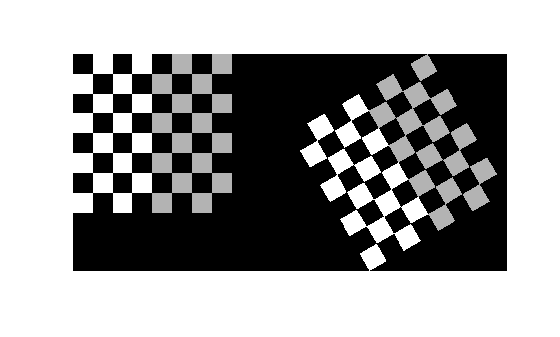Define some matching control points on the fixed image (the checkerboard) and moving image (the rotated checkerboard). You can define points interactively using the Control Point Selection tool.

fixedPoints = [41 41; 281 161];
movingPoints = [56 175; 324 160];

Create a geometric transformation that can be used to align the two images, returned as an affine2d geometric transformation object.

tform = fitgeotrans(movingPoints,fixedPoints,'NonreflectiveSimilarity')
tform =
affine2d with properties:

T: [3x3 double]
Dimensionality: 2

Use the tform estimate to resample the rotated image to register it with the fixed image. The regions of color (green and magenta) in the false color overlay image indicate error in the registration. This error comes from a lack of precise correspondence in the control points.

Jregistered = imwarp(J,tform,'OutputView',imref2d(size(I)));
figure
imshowpair(I,Jregistered)Recover angle and scale of the transformation by checking how a unit vector parallel to the x-axis is rotated and stretched.

u = [0 1];
v = [0 0];
[x, y] = transformPointsForward(tform, u, v);
dx = x(2) - x(1);
dy = y(2) - y(1);
angle = (180/pi) * atan2(dy, dx)
angle = 29.7686
scale = 1 / sqrt(dx^2 + dy^2)
scale = 1.0003

Input Arguments

collapse all

x- and y-coordinates of control points in the image you want to transform, specified as an m-by-2 matrix.

Example: movingPoints = [11 11; 41 71];

Data Types: double | single

x- and y- coordinates of control points in the fixed image, specified as an m-by-2 matrix.

Example: fixedPoints = [14 44; 70 81];

Data Types: double | single

Type of transformation, specified as one of the following: 'nonreflectivesimilarity', 'similarity', 'affine', or 'projective'. For more information, see Transformation Types.

Data Types: char | string

Degree of the polynomial, specified as the integer 2, 3, or 4.

Data Types: single | double | int8 | int16 | int32 | int64 | uint8 | uint16 | uint32 | uint64

Number of points to use in local weighted mean calculation, specified as a positive integer. n can be as small as 6, but making n small risks generating ill-conditioned polynomials.

Data Types: single | double | int8 | int16 | int32 | int64 | uint8 | uint16 | uint32 | uint64

Output Arguments

collapse all

Transformation, returned as a transformation object. The type of object depends on the transformation type. For example, if you specify the transformation type 'affine', then tform is an affine2d object. If you specify 'pwl', then tform is an image.geotrans.PiecewiseLinearTransformation2d object.

collapse all

Transformation Types

The table lists all the transformation types supported by fitgeotrans in order of complexity.

Transformation Type

DescriptionMinimum Number of Control Point PairsExample
'nonreflectivesimilarity'Use this transformation when shapes in the moving image are unchanged, but the image is distorted by some combination of translation, rotation, and scaling. Straight lines remain straight, and parallel lines are still parallel. 2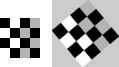'similarity'Same as 'nonreflectivesimilarity' with the addition of optional reflection.3'affine'Use this transformation when shapes in the moving image exhibit shearing. Straight lines remain straight, and parallel lines remain parallel, but rectangles become parallelograms.3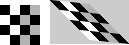'projective'Use this transformation when the scene appears tilted. Straight lines remain straight, but parallel lines converge toward a vanishing point.4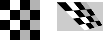'polynomial'Use this transformation when objects in the image are curved. The higher the order of the polynomial, the better the fit, but the result can contain more curves than the fixed image.

6 (order 2)

10 (order 3)

15 (order 4)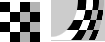'pwl'Use this transformation (piecewise linear) when parts of the image appear distorted differently.4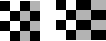'lwm'Use this transformation (local weighted mean) when the distortion varies locally and piecewise linear is not sufficient. 6 (12 recommended)Goshtasby, Ardeshir, "Piecewise linear mapping functions for image registration," Pattern Recognition, Vol. 19, 1986, pp. 459-466.

 Goshtasby, Ardeshir, "Image registration by local approximation methods," Image and Vision Computing, Vol. 6, 1988, pp. 255-261.